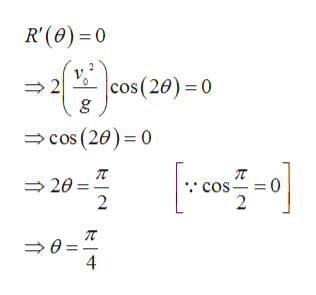# If we neglect air resistance, then the range of a ball (or any projectile) shot at an angle θ with respect to the x axis and with an initial velocity v0, is given byR(θ)=v02g sin(2θ)      for 0 ≤ θ ≤ π2 where g is the acceleration due to gravity (9.8 meters per second per second).For what value of θ is the maximum range attained? (Note that the answer is numerical, not symbolic.)θ =

Question
14 views

If we neglect air resistance, then the range of a ball (or any projectile) shot at an angle θ with respect to the x axis and with an initial velocity v0, is given by

R(θ)=

 v02 g

sin(2θ)      for 0 ≤ θ ≤

 π 2

where g is the acceleration due to gravity (9.8 meters per second per second).

For what value of θ is the maximum range attained? (Note that the answer is numerical, not symbolic.)

θ =

check_circle

Step 1

Given,

Step 2

On differentiating, we get

Step 3

Now computing the point where first...help_outlineImage TranscriptioncloseR'(0) 0 2 |cos (20) 0 go cos (20) 0 20 2 cos 0 = 2 4 fullscreen

### Want to see the full answer?

See Solution

#### Want to see this answer and more?

Solutions are written by subject experts who are available 24/7. Questions are typically answered within 1 hour.*

See Solution
*Response times may vary by subject and question.
Tagged in

### Derivative